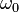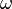# Ordinal number facts for kids

Kids Encyclopedia Facts

Ordinal numbers (or ordinals) are numbers that show something's order, for example: 1st, 2nd, 3rd, 4th, 5th.

Suppose a person has four different T-shirts, and then lays them in front of the person, from left to right.

• At the far left, there is the red T-shirt.
• Right of that is the blue one.
• Then there is the yellow one.
• And finally, at the far right is an orange T-shirt.

If the person then starts counting the shirts from the left, he would first see the red shirt. So the red shirt is the first T-shirt. The blue shirt is the second T-shirt. The yellow shirt is the third one, and the orange T-shirt is the fourth one.

The first, second, third, and fourth in this case are ordinal numbers. They result from the fact that the person has many objects, and they give them an order (hence 'ordinal'). The person then simply counts those objects, and gives the ordinal numbers to them.

In set theory, ordinals are also ordinal numbers people use to order infinite sets. An example is the set$\omega_0$ (or$\omega$ for short), which is the set containing all natural numbers (including 0). This is the smallest ordinal number that is infinite, and there are many more (such as$\omega$ + 1).

## Related pagesOrdinal number Facts for Kids. Kiddle Encyclopedia.